# Excel 2010 PMT (Payment) Function

Excel Payment (PMT) function is extremely useful when you need to know the monthly payment amount on full loan/credit payment, it actually evaluates amount to be paid monthly on the basis of credit amount, interest amount, and time. The basic parameters of this function are rate, nper (number of payments), pv (present value), and fv (future value), so if you need to calculate an amount which also considers these parameters, then this post will help.

To start off with, launch Excel 2010, and open the datasheet on which you want to apply PMT function. For instance, we have included a datasheet, containing fields; ID, Price, Interest Rate as shown in the screenshot below.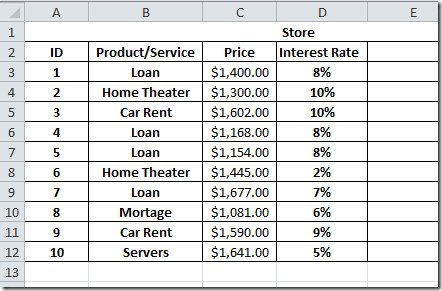Now we want to add a monthly payment, for this we need to set the rate we will be charging, so for this we assume that total payment will be paid by the client in 2 years (2*12=24). The Interest rate we are charging is 8%, so will add a new field by the name of Monthly Payment.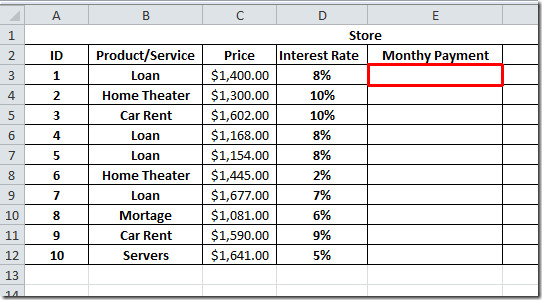Now we will add a PMT function that will evaluate how much each customer has to pay including interest.

The Syntax of PMT function is;

=PMT(rate, nper,pv,[fv],[type] )

In formula parameters, rate refers to, on which rate interest will be charged, nper stands for number of payments, pv refers to Present Value, you can also mention future value and type, but in our case we will leave these values.

So the rate we will be charging, is the sum of interest rate divided by 12 (number of months), clients need to pay us total amount + interest in 2 years so the nper value will be 12*2. The present value will be the price of the Product or Service.

So will we write the formula in this way;

=PMT(D3/12,2*12,C3)

The location D3 in the table refers to interest rate, and C3 in the datasheet refers to Price of Product/Service. When the function will be evaluated, it will yield \$63.32 as shown in the screenshot below.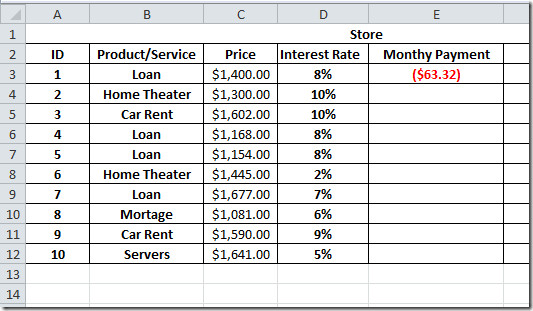Now apply it over the whole Monthly Payment field by dragging the plus sign at the end of it’s cell to the bottom of the column.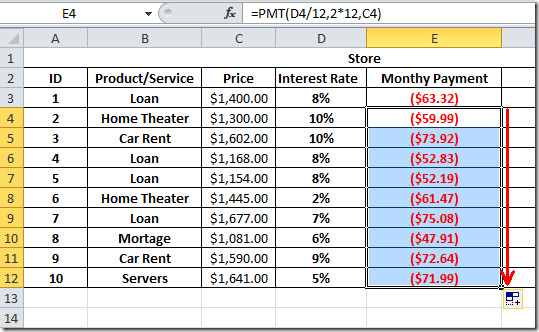In Excel, there is another way of applying PMT function, if you want to evaluate PMT function exclusively without creating fields, then select any cell where you want to show the result, navigate to Home tab, from Auto Sum options, click More Functions.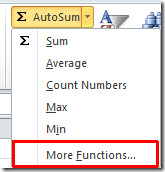You will reach Insert Function dialog, search for this function by providing PMT keyword, select the function name and click OK.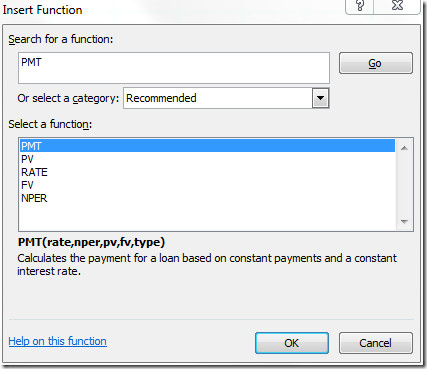Upon click, you will see the dialog asking you to enter values; Rate, nper, pv, fv, etc. Enter in the desired values as shown in the screenshot below, and click OK.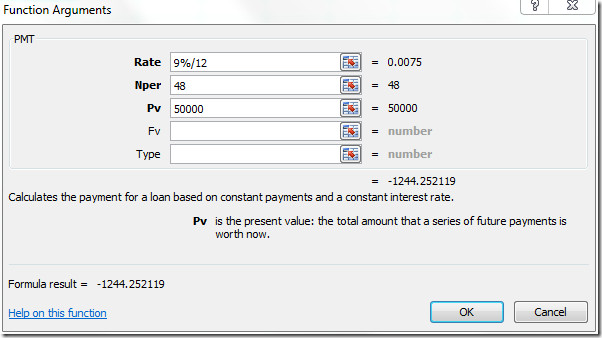Now you can see the result, Excel automatically puts-in the values in the selected cell.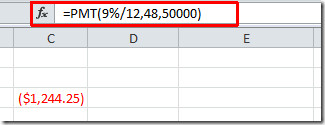You can also check out previously reviewed guides on HLOOKUP function & Using SmartArt in Excel.

1.patmelzer says:

Need to make an amortization schedule for daughter buying a car (I am the bank) making payments every OTHER month. any suggestions using pmt?

2.Lola says:

•Hareem says:

3.Sabrina says:

I have to figure out the payment amount with the customer making aw down payment on the cash price. How do I do that?

4.Jamal Adams says:

my ppmt funtion has the following variables PPMT(rate, per, nper, pv, [fv], [type]), where per is the month between 1 and nper…

5.Teris says: